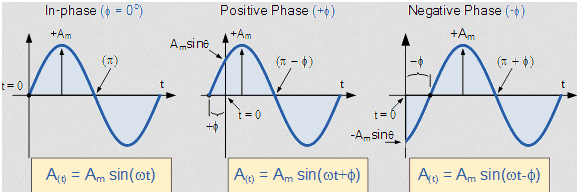# Phase Shift of a Sine Wave

• I
Summary:
Phase Shift
Hi,

I have created a sine wave with the following options:

1.) - changing the period/length in days of the sine wave (Cycle Length in Days)

2.) - calculating the start value of the "dummy" so that the sine wave always starts with -1 (Dummy Start at Cycle Trough) when the phase shift is set to "0".

3.) - calculating the phase shift, so that the sine wave moves a specific amount of time/days forward when I enter a positive value greater "0" in the "Phase Shift" input cell ("N2") or backward when I enter a negative value smaller "0" in the "Phase Shift" input cell ("N2").

Here is my Excel formula:

=+SIN((2*PI()*$H$2*B4)-$N$2)

I have attached my Excel file so you can easily see how my calculations work.

Now my problem/question:
I want make a phase shift of the sine wave with a specific amount of days e.g. 20 days forward or backward.

How can I calculate the phase shift value I enter in the cell "N2" with a formula so that the sine wave moves exactly e.g. 20 days forward or backward?

This formula should of course also work when I change the period/length in days of the sine wave (Cycle Length in Days) I enter in cell "F2".

Steven

#### Attachments

• Sine Wave Phase Shift.xlsx
56.5 KB · Views: 27

fresh_42
Mentor
A sine wave has the expression ##y(t)=A\cdot \sin(ct+p)##. ##A## is the amplitude, ##1/c## the period, and ##p## the phase shift.

•dextercioby
berkeman
Mentor
Summary:: Phase Shift

Now my problem/question:
I want make a phase shift of the sine wave with a specific amount of days e.g. 20 days forward or backward.

How can I calculate the phase shift value I enter in the cell "N2" with a formula so that the sine wave moves exactly e.g. 20 days forward or backward?Hi fresh_42 and berkeman,

I have understood the sine wave functionality and the issue with the positive and negative phase.

What I want is a formula to calculate the phase shift exactly as described in my post above. I have also attached an Excel file so you can see what I want.

Thanks

berkeman
Mentor
Summary:: Phase Shift

Now my problem/question:
I want make a phase shift of the sine wave with a specific amount of days e.g. 20 days forward or backward.
How many days is a full cycle of the sine wave? That corresponds to a full ##2\pi## cycle of the sine wave.
I have also attached an Excel file so you can see what I want.
We generally don't open Excel file attachments around here. It's too easy for there to be problems embedded in them. Instead, just attach a screenshot PDF or JPEG that shows the cells and formulas that you are asking about. Thanks.

Hi berkeman,

I have attached three screenshots:

1.) - with NO phase shift (in cell P2 the value 0.00 is entered)
2.) - with POSITIVE phase shift (in cell P2 the value 1.00 is entered)
3.) - with NEGATIVE phase shift (in cell P2 the value -1.00 is entered)

All screenshots uses 80 days for a full cycle of the sine wave. BUT the cycle length changes, it is NOT always 80 days it could be also 125 days, 95 days, 168 days, and so forth.

I hope this helps.

#### Attachments

•jim mcnamara
I have noticed that the screenshots are not very sharp and also can't easily be read so I have attached a PDF file.

#### Attachments

• Sine Waves with Phase Shifts.pdf
279.6 KB · Views: 20
Last edited:
RPinPA
Homework Helper
3.) - calculating the phase shift, so that the sine wave moves a specific amount of time/days forward when I enter a positive value greater "0" in the "Phase Shift" input cell ("N2") or backward when I enter a negative value smaller "0" in the "Phase Shift" input cell ("N2").

Here is my Excel formula:

=+SIN((2*PI()*$H$2*B4)-$N$2)

You want a different form of the sine wave, sin(k(d - d0))

A positive value of d0 will shift the sine wave left by d0 days, a negative value will shift it right.

I'm not sure which of those cell references corresponds to the day number d. But basically you want the shift to be grouped with the day, (d - d0).